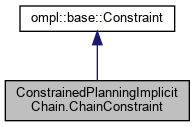ConstrainedPlanningImplicitChain.ChainConstraint Class Reference
Inheritance diagram for ConstrainedPlanningImplicitChain.ChainConstraint:[legend]

class  Wall

## Public Member Functions

def __init__ (self, links, obstacles=0, extra=1)

def function (self, x, out)

def jacobian (self, x, out)

def isValid (self, state)

def createSpace (self)

def getStartAndGoalStates (self)

def getProjection (self, space)

def dump (self, outfile)Public Member Functions inherited from ompl::base::Constraint
Constraint (const unsigned int ambientDim, const unsigned int coDim, double tolerance=magic::CONSTRAINT_PROJECTION_TOLERANCE)
Constructor. The dimension of the ambient configuration space as well as the dimension of the function's output need to be specified (the co-dimension of the constraint manifold). I.E., for a sphere constraint function in.

virtual void function (const State *state, Eigen::Ref< Eigen::VectorXd > out) const
Compute the constraint function at state. Result is returned in out, which should be allocated to size coDim.

virtual void function (const Eigen::Ref< const Eigen::VectorXd > &x, Eigen::Ref< Eigen::VectorXd > out) const =0
Compute the constraint function at x. Result is returned in out, which should be allocated to size coDim.

virtual void jacobian (const State *state, Eigen::Ref< Eigen::MatrixXd > out) const
Compute the Jacobian of the constraint function at state. Result is returned in out, which should be allocated to size coDim by ambientDim. Default implementation performs the differentiation numerically with a seven-point central difference stencil. It is best to provide an analytic formulation.

virtual void jacobian (const Eigen::Ref< const Eigen::VectorXd > &x, Eigen::Ref< Eigen::MatrixXd > out) const
Compute the Jacobian of the constraint function at x. Result is returned in out, which should be allocated to size coDim by ambientDim. Default implementation performs the differentiation numerically with a seven-point central difference stencil. It is best to provide an analytic formulation.

virtual bool project (State *state) const
Project a state state given the constraints. If a valid projection cannot be found, this method will return false. Even if this method fails, state will be modified.

virtual bool project (Eigen::Ref< Eigen::VectorXd > x) const
Project a state x given the constraints. If a valid projection cannot be found, this method will return false.

virtual double distance (const State *state) const
Returns the distance of state to the constraint manifold.

virtual double distance (const Eigen::Ref< const Eigen::VectorXd > &x) const
Returns the distance of x to the constraint manifold.

virtual bool isSatisfied (const State *state) const
Check whether a state state satisfies the constraints.

virtual bool isSatisfied (const Eigen::Ref< const Eigen::VectorXd > &x) const
Check whether a state x satisfies the constraints.

unsigned int getAmbientDimension () const
Returns the dimension of the ambient space.

unsigned int getManifoldDimension () const
Returns the dimension of the manifold.

unsigned int getCoDimension () const
Returns the dimension of the manifold.

void setManifoldDimension (unsigned int k)
Sets the underlying manifold dimension.

double getTolerance () const
Returns the tolerance of the projection routine.

unsigned int getMaxIterations () const
Returns the maximum number of allowed iterations in the projection routine.

void setTolerance (const double tolerance)
Sets the projection tolerance.

void setMaxIterations (const unsigned int iterations)
Sets the maximum number of iterations in the projection routine.

length

width

obstacles

extra

walls

cellSizes_

## Static Public Attributes

WALL_WIDTHProtected Attributes inherited from ompl::base::Constraint
const unsigned int n_
Ambient space dimension.

unsigned int k_
Manifold dimension.

double tolerance_
Tolerance for Newton method used in projection onto manifold.

unsigned int maxIterations_
Maximum number of iterations for Newton method used in projection onto manifold.

## Detailed Description

Definition at line 54 of file ConstrainedPlanningImplicitChain.py.

The documentation for this class was generated from the following file: